Next: Effective Area Up: In-flight Point Spread Previous: On-Axis PSF

#### Off-Axis PSF

The spatial resolution of the HRI off-axis   is dominated by the XRT PSF which degrades rapidly with increasing off-axis angle. Figures 5.7 and 5.8 display several off-axis pointings of HZ 43 and LMC X-1 and illustrate how the images change with off-axis angle. Figure 5.9 shows contours of the HZ 43 images. The images were first smoothed with a gaussian filter with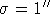(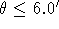),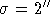(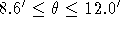), and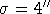(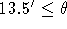). These images show that the PSF starts to degrade beyond 5' off-axis. Beyond 12' off-axis, the images become very asymmetrical. A central ``bar'' in the images well off-axis is perpendicular to a line connecting the optical axis and the image centroid.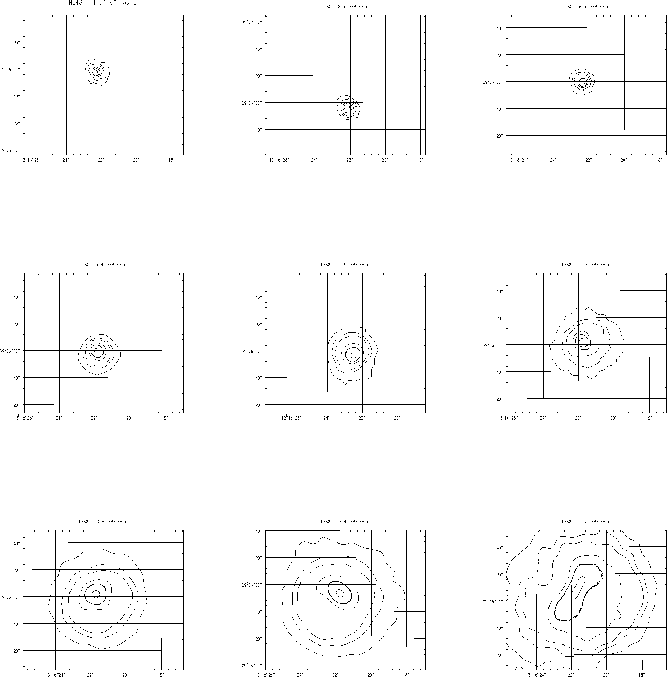Figure 5.9: Contour plots of the H 43 images. The contours are at 1, 5, 10, 30, 50, and 90% of the peak value, with the 50% contour level shown in bold.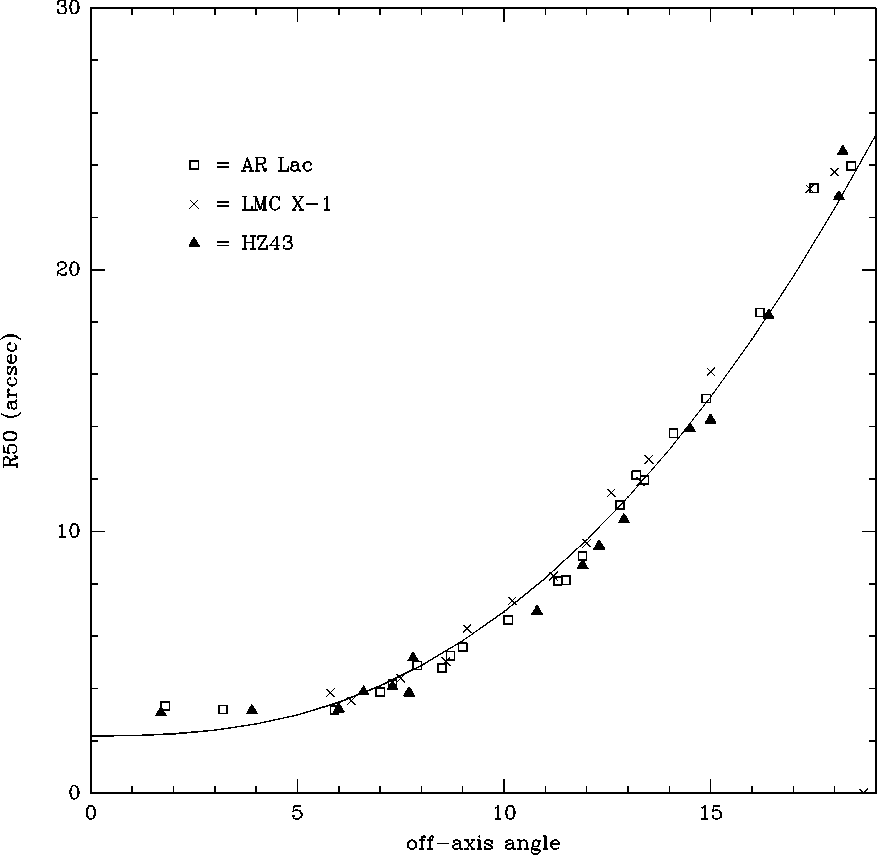Figure 5.10: The 50% power radius in images of H 43, LMC X-1, and AR Lac as a function of angle off-axis. The parametric expression given in the text is also shown for comparison.

The 50% power radius was determined for a series of off-axis pointings of HZ 43, AR Lac, and LMC X-1. The asymmetry at large angles has been ignored and the 50% power radius has been computed from radial binning about the image centroids. Figure 5.10 is a plot of the 50% power radius as a function of off-axis angle for these sources. The solid curve is the best-fit to the following parametric representation of the 50% power radius.whereis given in arc minutes, with the following values for the parameters: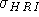= 0.74 arcsec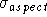= 1.0 arcsec= 1.3 arcsec a = 0.0205 b = 2.349

It is best to use a circle of radius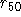when extracting counts of a source detected off-axis. The total net count rate (corrected for scattering) is then simply twice the net count rate within.

A parametric representation of the azimuthally averaged off-axis HRI PSF has also been derived which is a simple extension of the on-axis expression. To determine the off-axis PSF we generated surface brightness profiles for 8 observations of HZ 43, spanning a range from 2' to 16' off-axis. The peak surface brightness was used as the origin, since this generates a monotonically decreasing profile. The set of 8 HZ 43 surface brightness profiles were first fit to the on-axis PSF, treating the normalization and width of the two gaussians as free parameters (the photon statistics in these data were insufficient to fit the exponential term). We found that the best fit values of the smaller gaussian were essentially independent of the angle off-axis. We then refit the set of 8 profiles treating only the width of the broader gaussian as a free parameter. The best fit values of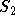are well parameterized by a cubic polynomial in the off-axis angle, and are given by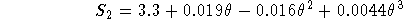where theta is the angle off-axis in arc minutes. Substituting this expression into the on-axis HRI PSF gives the general off-axis representation.

NB: We do not force this expression to reproduce the on-axis value given above. The fact that this expression gives= 3.3 for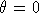, compared to the on-axis value of 4.0 given above, reflects the variation in aspect quality from observation to observation.

Users should note that point sources observed off-axis  exhibit significant amounts of nonazimuthal symmetry. The above expression is useful for determining scattering corrections and for use in detect algorithms, but should not be used for image deconvolution. There are a large number of sequences in the PROS calibration directory `xrcal` containing several objects observed at different angles off-axis. The actual HRI data should be used for any two-dimensional image deconvolution.Next: Effective Area Up: In-flight Point Spread Previous: On-Axis PSF

If you have problems/suggestions please send mail to rosat_svc@mpe-garching.mpg.de